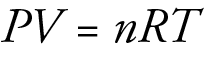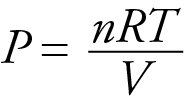﻿ Changing Volumes and Equilibrium

#The Effect of Changing Volume on Equilibrium Systems

Many industrial gas-phase reactions are run at very high gas pressure created by compressing gases originally at room pressure to a much smaller volume. To see why this is done, let’s take a closer look at the effect of changes in volume on gas-phase reactions.

If all other factors remain constant, changing the volume occupied by a gas will change its concentration, and therefore, change the rate at which it reacts with other substances. For example, for the following reaction, decreasing the volume occupied by the gases by half will double their concentrations.

N2O4(g)2NO2(g)

Doubling the concentration of N2O4 doubles the forward rate of reaction. In contrast, because there are two moles of NO2 involved in the reverse reaction, doubling the concentration of NO2 leads to four times the rate of the reverse reaction. The first important point here is that changing the volume occupied by a gas-phase reaction system leads to change in both the forward and reverse reaction rates. The second important point is that the effect on these two rates may not be the same. If the effect on the rates is different, equilibrium will be disrupted, and the reaction will shift toward more products or more reactants. In our case, because the reverse rate is increased more than the forward rate, the system will shift toward more reactants.

In general, decreased volume and increased concentration will lead to an increase in both the forward and reverse rates, but it will cause a greater increase in the rate (forward or reverse) whose “reactants” have more moles of gas. (Remember, the “products” are the “reactants” of the reverse reaction.) Thus decreased volume for a gas-phase reaction will shift the system toward the side of the reaction with the fewest moles of gas. For example, decreased volume and therefore increased concentration of both reactants and products for the following reaction at equilibrium will shift the system toward more products.

CO(g)  +  Cl2(g)COCl2(g)

2 moles1 mole

The decreased volume only disrupts the equilibrium if the moles of gaseous products and moles of gaseous reactants are unequal. If there are an equal number of moles of gaseous substances on both sides of the arrow, the change in volume has an equal effect on the concentrations of reactants and of products. Thus, it has an equal effect on the forward and reverse rates, and the system remains at equilibrium. For example, a change in volume does not disrupt the equilibrium for the reaction that forms hydrogen gas.

CO(g)  +  H2O(g)CO2(g)  +  H2(g)

2 moles2 moles

EXAMPLE 1 - Predicting the Effect of Changing Volume on Gas-Phase Reactions: Predict whether a decrease in the volume of the container will drive an equilibrium system for each reaction toward more products, toward more reactants, or neither. Explain your answers.

a. NH3(g)  +  2O2(g)HNO3(l)  +  H2O(l)

b. CO2(g)  +  CF4(g)2COF2(g)

c. C(s)  +  H2O(g)CO(g)  +  H2(g)

Solution:

a. Decreased volume shifts the system to the side of the reaction that has fewer moles of gas. For this reaction, there are three moles of gaseous reactants, and no moles of gaseous products, so the shift will be toward products.

b. This reaction has the same number of moles of gaseous reactants and products, so changing the volume for the reaction will not shift the system either way. Neither reactants nor products are favored.

c. You need to be careful with this one. Although there are the same number of moles of reactants and products, one of the reactants is a solid. Thus, there are fewer moles of gaseous reactants than gaseous products, so decreased volume shifts the system toward reactants.

By considering the effect of changing volume on gas pressure, we can also use Le Chatelier’s principle to help us predict the effect of changing volume on gas phase reactions. Decreased volume leads to an increase in pressure. For an ideal gas, cutting the volume in half leads to doubling the gas pressure.Le Chatelier’s principle helps us decide that decreasing the volume for the following reaction, therefore increasing the total gas pressure, will lead to a shift in the system to counteract this change, that is, to decrease the gas pressure.

N2O4(g)2NO(g)

There are fewer moles of gaseous reactants than gaseous products, if the system shifts toward reactants, the gas pressure will decrease. The table below provides a general summary of how Le Chatelier’s principle can be used to predict shifts in equilibrium systems.

Summary of the Volume-Change-Shifts in Equilibrium Predicted by Le Chatelier’s Principle

 Reaction Cause of Disruption To Counteract Change Direction of Shift More moles of gaseous products than reactants Decrease volume (and increase pressure) Decrease pressure To reactants More moles of gaseous products than reactants Increase volume (and decrease pressure) Increase pressure To products More moles of gaseous reactants than products Decrease volume (and increase pressure) Decrease pressure To products More moles of gaseous reactants than products Increase volume (and decrease pressure) Increase pressure To reactants Equal moles of gaseous reactants and products Decrease volume (and increase pressure) No effect No Shift Equal moles of gaseous reactants and products Increase volume (and increase pressure) No effect No Shift

EXAMPLE 2 - Predicting the Effect of Disruptions on Equilibrium: Ammonia gas, which is used to make fertilizers and explosives, is made from the reaction of nitrogen gas and hydrogen gas. The forward reaction is exothermic. Consider a system in which the gases are compressed to a volume that is small enough to yield a total pressure of about 300 atm. (This is a typical pressure for the industrial production of ammonia.) Predict whether this change in an equilibrium system of nitrogen, hydrogen, and ammonia will shift the system to more products, to more reactants, or neither. Explain each answer in two ways, (1) by applying Le Chatelier’s principle and (2) by describing the effect of the change on the forward and reverse reaction rates.

N2 (g)  +  3H2(g)2NH3(g)  +  92.2 kJ

Solution:

(1) Using Le Chatelier's Principle, we predict that the system shifts to partially counteract the increase in pressure. Because there are four moles of gaseous reactants for every two moles of gaseous products, the system will shift toward more products. Decreasing the moles of gas will decrease the overall pressure.

(2) Decreased volume with constant moles of gas leads to an increase in the concentration (mol/L) of all of the reactants and products. This will increase the rate of both the forward and reverse reactions, but it will increase the rate of the reaction that involves the greater number of moles of gas more than the rate of the reaction that involves fewer moles of gas. In our reaction, the forward reaction (with two times the moles of gas) is increased more than the reverse reaction (with half as many moles of gas). This leads to a shift toward more products.

Chiral Publishing Company